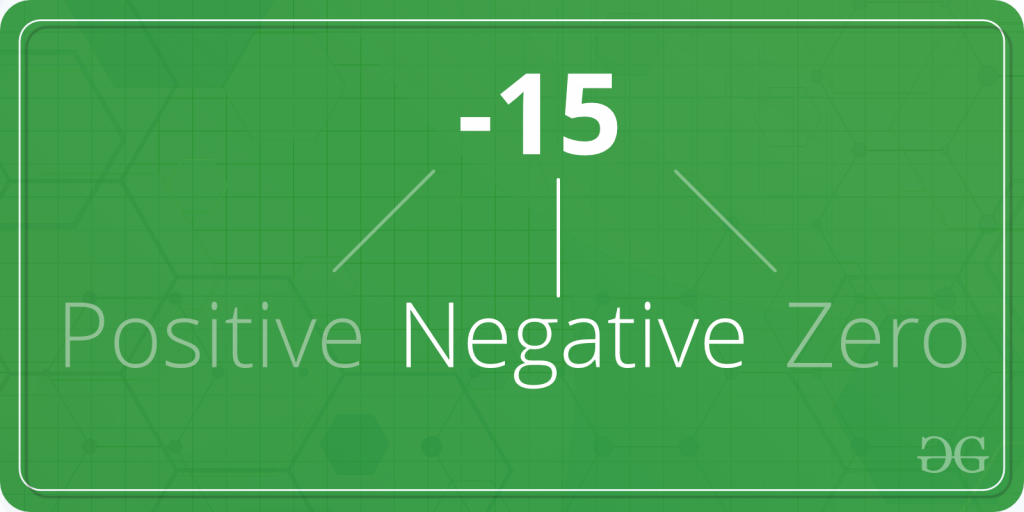# C program to Check Whether a Number is Positive or Negative or Zero

• Difficulty Level : Basic
• Last Updated : 28 Jun, 2022

Given a number A. The task is to check whether A is positive, negative or zero.Examples:

```Input: A = 2
Output: 2 is positive

Input: A = -554
Output: -554 is negative```

In the below program, to find whether A is positive, negative or zero; first the number is taken as input from the user using scanf in, and then A is checked for positive usingstatement andandoperators. Below is the C program to find whether a number is positive, negative or zero.

## C

 `#include ` `int` `main()``{``    ``int` `A;` `    ``printf``(``"Enter the number A: "``);``    ``scanf``(``"%d"``, &A);` `    ``if` `(A > 0)``        ``printf``(``"%d is positive."``, A);``    ``else` `if` `(A < 0)``        ``printf``(``"%d is negative."``, A);``    ``else` `if` `(A == 0)``        ``printf``(``"%d is zero."``, A);` `    ``return` `0;``}`

Output:

```Enter the number A =: -54
-54 is negative.```

Time Complexity: O(1)

Auxiliary Space: O(1)

My Personal Notes arrow_drop_up